#### Triangulation

One alternative to the vertical decomposition is to perform a triangulation, which yields a simplicial complex over. Figure 6.16 shows an example. Sinceis an open set, there are no 0-cells. Each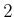-simplex (triangle) has either one, two, or three faces, depending on how much of its boundary is shared with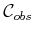. A roadmap can be made by connecting the samples for-cells and-cells as shown in Figure 6.17. Note that there are many ways to triangulatefor a given problem. Finding good triangulations, which for example means trying to avoid thin triangles, is given considerable attention in computational geometry [129,264,302].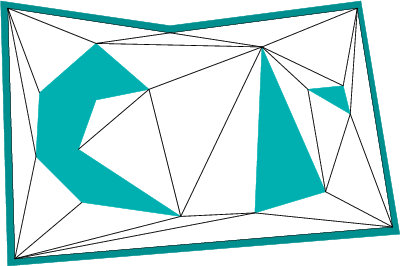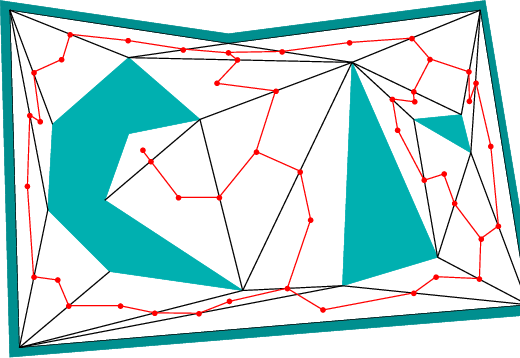How can the triangulation be computed? It might seem tempting to run the vertical decomposition algorithm of Section 6.2.2 and split each trapezoid into two triangles. Even though this leads to triangular cells, it does not produce a simplicial complex (two triangles could abut the same side of a triangle edge). A naive approach is to incrementally split faces by attempting to connect two vertices of a face by a line segment. If this segment does not intersect other segments, then the split can be made. This process can be iteratively performed over all vertices of faces that have more than three vertices, until a triangulation is eventually obtained. Unfortunately, this results in an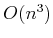time algorithm because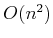pairs must be checked in the worst case, and each check requires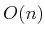time to determine whether an intersection occurs with other segments. This can be easily reduced to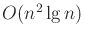by performing radial sweeping. Chapter 3 of  presents an algorithm that runs in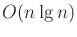time by first partitioninginto monotone polygons, and then efficiently triangulating each monotone polygon. Ifis simply connected, then, surprisingly, a triangulation can be computed in linear time . Unfortunately, this algorithm is too complicated to use in practice (there are, however, simpler algorithms for which the complexity is close to; see  and the end of Chapter 3 of  for surveys).

Steven M LaValle 2020-08-14